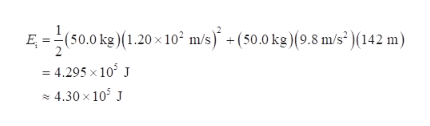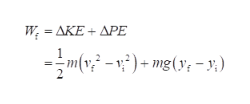# A 50.0 - kg projectile is fired at an angle of 30.0° above the horizontalwith an initial speed of 1.20 x 102 m/s from the top ofa cliff 142 m above level ground, where the ground is taken tobe y = 0. (a) What is the initial total mechanical energy of theprojectile? (b) Suppose the projectile is traveling 85.0 m/s atits maximum height of y = 427 m. How much work has beendone on the projectile by air friction? (c) What is the speed ofthe projectile immediately before it hits the ground if air frictiondoes one and a half times as much work on the projectilewhen it is going down as it did when it was going up?

Question
3 views

A 50.0 - kg projectile is fired at an angle of 30.0° above the horizontal
with an initial speed of 1.20 x 102 m/s from the top of
a cliff 142 m above level ground, where the ground is taken to
be y = 0. (a) What is the initial total mechanical energy of the
projectile? (b) Suppose the projectile is traveling 85.0 m/s at
its maximum height of y = 427 m. How much work has been
done on the projectile by air friction? (c) What is the speed of
the projectile immediately before it hits the ground if air friction
does one and a half times as much work on the projectile
when it is going down as it did when it was going up?

check_circle

Step 1

(a) Write the expression for initial mechanical energy

Step 2

Substitute the valueshelp_outlineImage TranscriptioncloseE, =(50.0 kg)(1.20 x10° m/s) +(50.0 kg)(9.8 m/s² )(142 m) : 4.295 x 10 J 4.30 x 10° J fullscreen
Step 3

(b) Write the expression for work done ...help_outlineImage TranscriptioncloseΔΚE+ ΔΡΕ W; = AKE + %3D --m(v - ) + mg(y; - 3:) fullscreen

### Want to see the full answer?

See Solution

#### Want to see this answer and more?

Solutions are written by subject experts who are available 24/7. Questions are typically answered within 1 hour.*

See Solution
*Response times may vary by subject and question.
Tagged in
SciencePhysics

### Work,Power and Energy